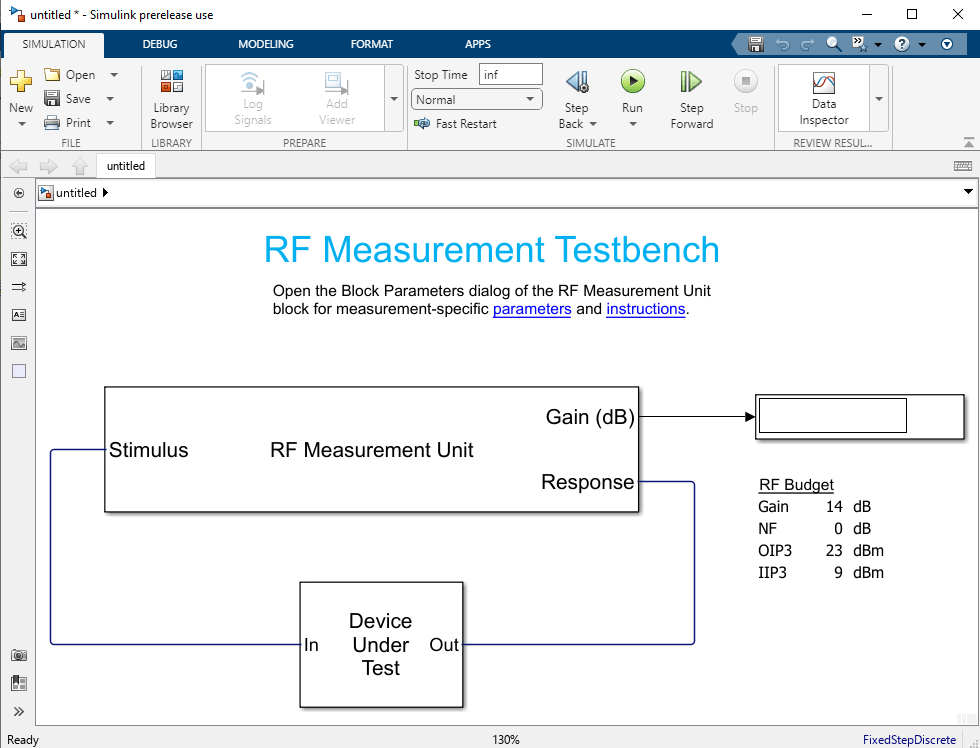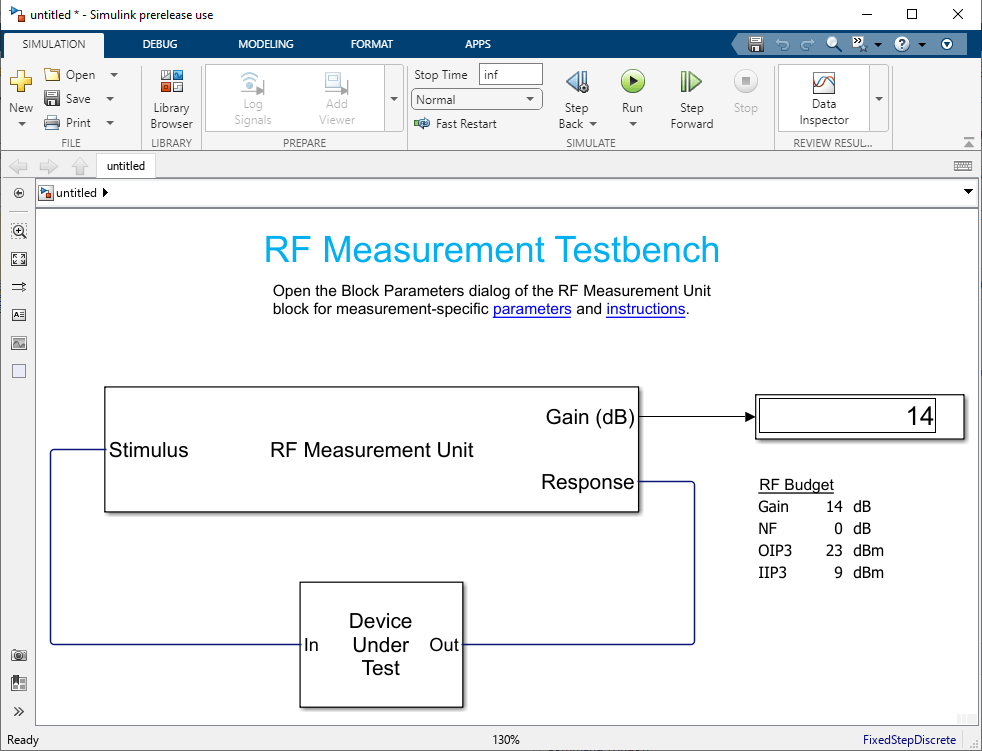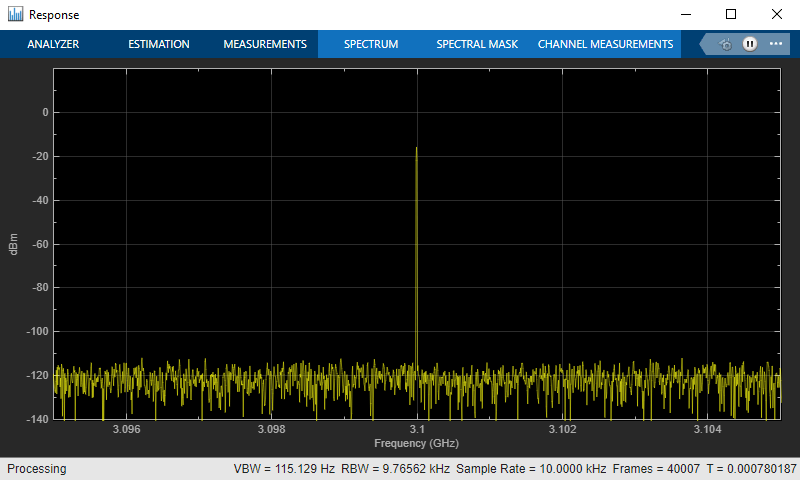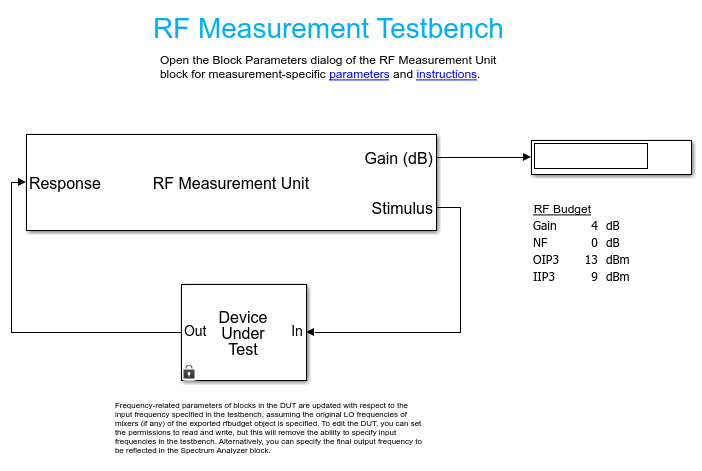# exportTestbench

Export RF budget object to RF Blockset measurement testbench model

## Syntax

``exportTestbench(rfobj)``
``exportTestbench(rfobj,Library='IdealizedBaseband')``
``sys = exportRFBlockset(___)``

## Description

example

````exportTestbench(rfobj)` exports the RF budget object `robj` to a measurement testbench model in the RF Blockset™ Circuit Envelope library environment. You can then use the model to measure the cumulative gain, input second-order intercept point (IIP2), output second-order intercept point (OIP2), input third-order intercept point (IIP3), output third-order intercept point (OIP3), noise figure, noise floor, and DC offsets.```

example

````exportTestbench(rfobj,Library='IdealizedBaseband')` exports the RF budget object to a measurement testbench model in the RF Blockset Idealized Baseband library environment. You can then use the model to measure gain, intercept points, and noise floor. Idealized baseband simulation provides shorter simulation time because the simulation assume perfect impedance matching and a nominal impedance of 1 ohm in the RF system. (since R2023a)```
````sys = exportRFBlockset(___)` returns the system name.```

Note

The `exportTestbench` function requires a license for RF Blockset and DSP System Toolbox™.

## Examples

expand all

Create an amplifier with a gain of 4 dB.

`a = amplifier(Gain=4);`

Create a modulator with an OIP3 of 13 dBm.

`m = modulator(OIP3=13);`

Create an RF element with a gain of 10 dB.

`r = rfelement(Gain=10);`

Calculate the RF budget of a series of RF elements at an input frequency of 2.1 GHz, an available input power of –30 dBm, and a bandwidth of 10 MHz.

`rfobj = rfbudget([a m r],2.1e9,-30,10e6)`
```rfobj = rfbudget with properties: Elements: [1x3 rf.internal.rfbudget.RFElement] InputFrequency: 2.1 GHz AvailableInputPower: -30 dBm SignalBandwidth: 10 MHz Solver: Friis AutoUpdate: true Analysis Results OutputFrequency: (GHz) [ 2.1 3.1 3.1] OutputPower: (dBm) [ -26 -26 -16] TransducerGain: (dB) [ 4 4 14] NF: (dB) [ 0 0 0] IIP2: (dBm) [] OIP2: (dBm) [] IIP3: (dBm) [ Inf 9 9] OIP3: (dBm) [ Inf 13 23] SNR: (dB) [73.98 73.98 73.98] ```

Create the measurement testbench from the RF budget object.

```exportTestbench(rfobj) ```Select Run to simulate your testbench.The response will be displayed when you run the testbench.Since R2023a

Create an amplifier with a gain of 4 dB.

```a = amplifier(Gain=4); ```

Create a modulator with an OIP3 of 13 dBm.

```m = modulator(OIP3=13); ```

Calculate the RF budget of a series of RF elements at an input frequency of 2.1 GHz, an available input power of – 30 dBm, and a bandwidth of 10 MHz.

```rfobj = rfbudget([a m ],2.1e9,-30,10e6); ```

Export the RF budget object to an RF Blockset measurement testbench model created using Idealized Baseband library blocks.

```exportTestbench(rfobj,Library='IdealizedBaseband') ```Use the RF Measurement testbench to verify the cumulative gain, noise figure or noise floor, and nonlinearity (IP3) values while taking advantage of the shorter simulation times of the Idealized Baseband library by assuming perfect impedance matching and a nominal impedance of 1 ohm.Modify the RF system in the device under test for further analysis

## Input Arguments

expand all

RF budget object, specified as an `rfbudget` object.

Note

You cannot export an RF budget object to an RF Blockset Idealized Baseband measurement testbench model if one of the elements in the object is an `rfelement`. (since R2023a)

## Version History

Introduced in R2017a

expand all